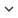ENGArticle
Article 421
Author/s
• Constantino A. García, Abraham Otero, Paulo Félix, Jesús Presedo, David G. Márquez
DOI
Source
• Physica D-nonlinear phenomena, 2018 - Q1

# Simultaneous estimation of deterministic and fractal stochastic components in non-stationary time series

In the past few decades, it has been recognized that 1 / f fluctuations are ubiquitous in nature. The most widely used mathematical models to capture the long-term memory properties of 1 / f fluctuations have been stochastic fractal models. However, physical systems do not usually consist of just stochastic fractal dynamics, but they often also show some degree of deterministic behavior. The present paper proposes a model based on fractal stochastic and deterministic components that can provide a valuable basis for the study of complex systems with long-term correlations. The fractal stochastic component is assumed to be a fractional Brownian motion process and the deterministic component is assumed to be a band-limited signal. We also provide a method that, under the assumptions of this model, is able to characterize the fractal stochastic component and to provide an estimate of the deterministic components present in a given time series. The method is based on a Bayesian wavelet shrinkage procedure that exploits the self- similar properties of the fractal processes in the wavelet domain. This method has been validated over simulated signals and over real signals with economical and biological origin. Real examples illustrate how our model may be useful for exploring the deterministic–stochastic duality of complex systems, and uncovering interesting patterns present in time series.
Keywords: 1 / f noise, Fractional Brownian motion, Bayesian estimation, Wavelet transform